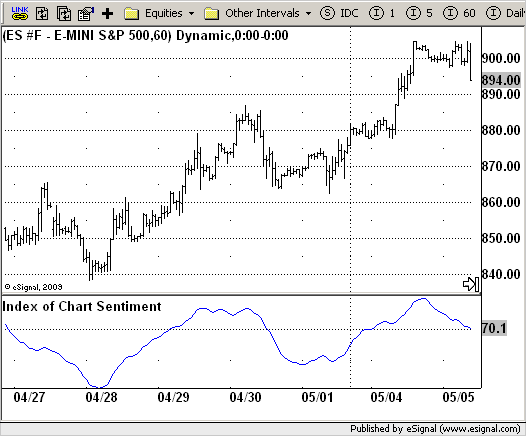# Index of Chart Sentiment (ICS)

ICE Data Services -

File Name: ICS.efs

Description:
Index of Chart Sentiment (ICS)

Formula Parameters:
BBlength : 55
Deviations : 0.5

Notes:
The main problem of technical indicators is the identifying a trend in its
early stages.
To effectively do this, Mr. Likhovidov created an indicator called the Index
of Chart Sentiment (ICS). The ICS could reach very high levels and this corresponds
to an overbought market; very low values of the index correspond to an oversold market.
When the index moves from lower to higher levels (period of bullish sentiment of the
market) the dominant movement of the chart should be to the upside.
The algorithm for calculating the CandleCode involves a long-period analysis of the
chart. But this analysis does not consist of the usual smoothing and therefore does
not result in any lag.
in recent S&C issue.

ICS.efsEFS Code:

```/*********************************
Provided By:
eSignal (Copyright c eSignal), a division of Interactive Data
Formula Script (EFS) is for educational purposes only and may be
modified and saved under a new file name.  eSignal is not responsible
for the functionality once modified.  eSignal reserves the right
to modify and overwrite this EFS file with each new release.

Description:
Index of Chart Sentiment (ICS)

Version:            1.0  05/13/2009

Formula Parameters:                     Default:
BBlength                            55
Deviations                          0.5

Notes:
The main problem of technical indicators is the identifying a trend in its
early stages.
To effectively do this, Mr. Likhovidov created an indicator called the Index
of Chart Sentiment (ICS). The ICS could reach very high levels and this corresponds
to an overbought market; very low values of the index correspond to an oversold market.
When the index moves from lower to higher levels (period of bullish sentiment of the
market) the dominant movement of the chart should be to the upside.
The algorithm for calculating the CandleCode involves a long-period analysis of the
chart. But this analysis does not consist of the usual smoothing and therefore does
not result in any lag.
in recent S&C issue.
**********************************/
var fpArray = new Array();
var bInit = false;

function preMain() {
setPriceStudy(false);
setShowTitleParameters( false );
setStudyTitle("Index of Chart Sentiment");
setCursorLabelName("ICS",0);
setDefaultBarFgColor(Color.blue,0);
var x = 0;
fpArray[x] = new FunctionParameter("BBlength", FunctionParameter.NUMBER);
with(fpArray[x++]) {
setLowerLimit(1);
setDefault(55);
}
fpArray[x] = new FunctionParameter("Deviations", FunctionParameter.NUMBER);
with(fpArray[x++]) {
setLowerLimit(0.001);
setDefault(0.5);
}
}

var xICS = null;

function main(BBlength, Deviations) {
var nBarState = getBarState();
var nICS = 0;
if (nBarState == BARSTATE_ALLBARS) {
if(BBlength == null) BBlength = 55
if(Deviations == null) Deviations = 0.5
}
if (bInit == false) {
xICS = ema(24, ema(24, efsInternal("Calc_ICS", BBlength, Deviations)));
bInit = true;
}
nICS = xICS.getValue(0);
if (nICS == null) return;
return nICS;
}

var xOpen = null;
var xClose = null;
var xBody = null;
var	xThTop_Body = null;
var	xThBot_Body = null;
var	xThTop_Ushd = null;
var	xThBot_Ushd = null;
var	xThTop_Lshd = null;
var	xThBot_Lshd = null;
var bSecondInit = false;

function Calc_ICS(BBlength, Deviations) {
var Body = 0;
var ThBot_Body = 0;
var ThTop_Body = 0;
var ThBot_Ushd = 0;
var ThTop_Ushd = 0;
var ThBot_Lshd = 0;
var ThTop_Lshd = 0;
var ColorCode = 0;
var BodyCode = 0;
var UshdCode = 0;
var LshdCode = 0;
var nRes = 0;

if (bSecondInit == false) {
xOpen = open();
xClose = close();
xBody = efsInternal("GetCandleValue", xOpen, xClose);
xThTop_Body = upperBB(BBlength, Deviations, xBody);
xThBot_Body = lowerBB(BBlength, Deviations, xBody);
bSecondInit = true;
}
Body = xBody.getValue(0);
ThTop_Body = xThTop_Body.getValue(0);
ThBot_Body = xThBot_Body.getValue(0);
ThTop_Ushd = xThTop_Ushd.getValue(0);
ThBot_Ushd = xThBot_Ushd.getValue(0);
ThTop_Lshd = xThTop_Lshd.getValue(0);
ThBot_Lshd = xThBot_Lshd.getValue(0);
if (ThBot_Lshd == null) return;
if(xClose.getValue(0) >= xOpen.getValue(0)){
ColorCode = 64;
if(xOpen.getValue(0) == xClose.getValue(0))
BodyCode = 0;
else if(Body < ThBot_Body)
BodyCode = 16;
else if(Body < ThTop_Body)
BodyCode = 32;
else
BodyCode = 48;
}
else{
ColorCode = 0;
if(Body < ThBot_Body)
BodyCode = 32;
else if(Body < ThTop_Body)
BodyCode = 16;
else
BodyCode = 0;
}
UshdCode = 0;
UshdCode = 4;
UshdCode = 8;
else
UshdCode = 12;

LshdCode = 3;
LshdCode = 2;
LshdCode = 1;
else
LshdCode = 0;

nRes = ColorCode + BodyCode + UshdCode + LshdCode;
return nRes;
}

function GetCandleValue(xOpen, xClose) {
var Body = Math.abs(xOpen.getValue(0) - xClose.getValue(0));
var UpperShadow = high(0) - Math.max(xOpen.getValue(0),xClose.getValue(0));
var LowerShadow = Math.min(xOpen.getValue(0),xClose.getValue(0)) - low(0);